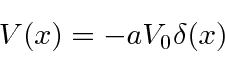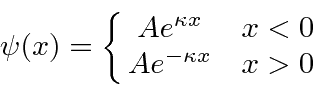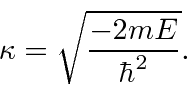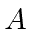## The Delta Function Potential *

Take a simple, attractive delta function potential and look for the bound states.These will have energy less than zero so the solutions arewhereThere are only two regions, above and below the delta function. We don't need to worry about the one point at- the two solutions will match there. We have already made the wave function continuous atby using the same coefficient,, for the solution in both regions.We now need to meet the boundary condition on the first derivative at. Recall that the delta function causes a known discontinuity in the first derivative.Putting in the formula forin terms of the energy.There is only one energy for which we can satisfy the boundary conditions. There is only one bound state in an attractive delta function potential.

Jim Branson 2013-04-22